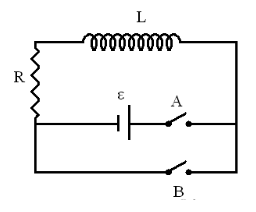# Problem: An RL circuit is shown on the right. L = 2.2 J, R = 258 Ω, ε = 4.5 VSwitch A is closed at t = 0. Part (a) After a long time, when the current reaches its steady value, open switch A and close switch B at the same time. Count this moment as t = 0. Express the current in the circuit, I, as a function of time in terms of L, R and e.Part (b) Now what is the direction of the current I, counterclockwise or clockwise?

###### FREE Expert Solution

Part (a)

The current in the circuit is given by:

i(t) = i0e(-R/L)t###### Problem Details

An RL circuit is shown on the right. L = 2.2 J, R = 258 Ω, ε = 4.5 VSwitch A is closed at t = 0.

Part (a) After a long time, when the current reaches its steady value, open switch A and close switch B at the same time. Count this moment as t = 0. Express the current in the circuit, I, as a function of time in terms of L, R and e.

Part (b) Now what is the direction of the current I, counterclockwise or clockwise?